Next: FEWER EQUATIONS THAN UNKNOWNS Up: Data modeling by least Previous: MORE EQUATIONS THAN UNKNOWNS

# WEIGHTS AND CONSTRAINTS

It often happens that some observations are considered more reliable than others. One may desire to weight the more reliable data more heavily in the calculation. In other words, we may multiply the ith equation by a weight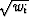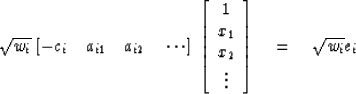(28)
Now the weighted sum-squared error will be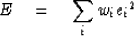(29)
Following the method of the last section, it is easy to show that the x which minimizes the weighted error E of (29) is the x which satisfies the simultaneous equations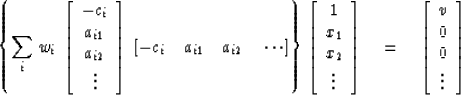(30)
Choice of a set of weights is often a rather subjective matter. However, if data are of uneven quality, it cannot be avoided. Omitting w is equivalent to choosing it equal to unity.

A case of common interest is where some equations should be solved exactly. Such equations are called constraint equations. Constraint equations often arise out of theoretical considerations so they may, in principle, not have any error. The rest of the equations often involve some measurement. Since the measurement can often be made many times, it is easy to get a lot more equations than unknowns. Since measurement always involves error, we then use the method of least squares to minimize the average error. In order to be certain that the constraint equations are solved exactly, one could use the trick of applying very large weight factors to the constraint equations. A problem is that very large'' is not well defined. A weight equal 1010 might not be large enough to guarantee the constraint equation is satisfied with sufficient accuracy. On the other hand, 1010 might lead to disastrous round-off when solving the simultaneous equations in a computer with eight-digit accuracy. The best approach is to analyze the situation theoretically for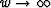.

An example of a constraint equation is that the sum of the xi equals M. Another constraint would be x1 = x2. Arranged in a matrix, these two constraint equations are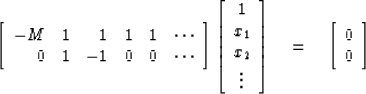(31)
We write a general set of k constraint equations as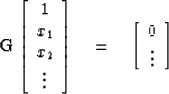(32)
Minimizing the error asof the equations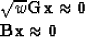is algebraically similar to minimizing the error of Bx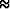0. The rows of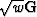are just like some extra rows for B. The resulting equation for x is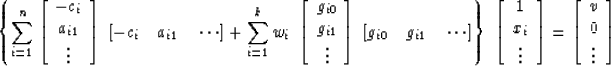(33)
Now we will take all the wi to equal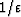and we will lettend to zero. Also let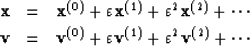(34) (35)
With this, (33) may be written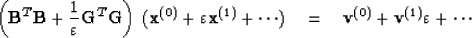(36)
Identify coefficients of powers of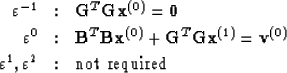(37) (38)
Equation (37) is m equations in m unknowns. It will automatically be satisfied if the k equations in (32) are satisfied. Equation (38) appears to involve the m unknowns in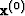plus m more unknowns in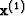. In fact, we do not need;the k unknowns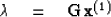(39)
will suffice.

Arranging (38) and (32) together and dropping superscripts, we get a square matrix in m + k unknowns.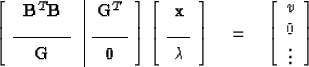(40)

Equation (40) is now a simultaneous set for the unknowns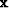and. It might also be thought of as the solution to the problem of minimizing the quadratic form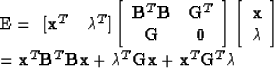and since we can always transpose a scalar,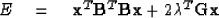(41)

According to the method of Lagrange multipliers, one may minimize a quadratic form subject to constraints by minimizing instead a sum of the quadratic form plus constraint terms where each constraint term is the product of a constraint equation multiplied by a Lagrange multiplier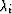. This is precisely what we have in (41), and the solution is given by (40). Lagrange multipliers frequently arise in connection with integral equations. The concept is readily transformed to matrices merely by approximating integration by summation.

## EXERCISES:

1. In determining a density vs. depth profile of the earth one might minimize the squared difference between some theoretical quantities (say, the frequencies of free oscillation) and the observed quantities. By astronomical means, total mass and moment of inertia of the earth are very well known. If the earth is divided into arbitrarily thin shells of equal thickness, what are the two astronomical constraint equations on the layer densities pi? If the least-squares problem is nonlinear (as it often is) it may be linearized by assuming that a given set of densities pi is a good guess which satisfies the constraints and doing least squares for the perturbation dpi. What are the constraint equations on dpi?Next: FEWER EQUATIONS THAN UNKNOWNS Up: Data modeling by least Previous: MORE EQUATIONS THAN UNKNOWNS
Stanford Exploration Project
10/30/1997